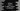# How to check if a tuple contains an element in Python## How to check if a tuple contains an element in Python:

In this post, we will learn how to check if a python tuple contains an element. There are different ways to do that. We can either iterate through its items or we can use if-else to do that in a simple way.

In this post, I will show you how to do that with two different examples.

## Method 1: By using a loop:

Let’s try it by using a loop. We will iterate through each items of the tuple one by one and compare it with the given value. If any value in the tuple is equal to the given value, it will return True. Else, it will return False.

Below is the complete program:

``````def contains(tuple, given_char):
for ch in tuple:
if ch == given_char:
return True
return False

given_tuple = ('a', 'b', 'c', 'd', 'e', 'f', 'g', 'h')

char = input('Enter a character to find: ')

if contains(given_tuple, char):
print('It is in the tuple')
else:
print('It is not in the tuple')``````

Here,

• contains method is used to check if a character is in a given tuple or not.
• This method returns one boolean value. Based on its result, we are printing one message.
• This method takes one tuple and one character as parameters. It iterates through the characters of the tuple and if it finds any character equal to the provided character, it returns True. Else, it returns False if the character is not found in the tuple.
• It takes one character as input from the user and calls contains method to check if that character is in the tuple or not.

If you run this program, it will give output as like below:

``````Enter a character to find: i
It is not in the tuple

Enter a character to find: e
It is in the tuple``````

## Method 2: By using if..not:

We can also quickly check if an element is in a tuple or not by using if..not check. Let me change the above program to use if..not:

``````def contains(tuple, given_char):
if given_char in tuple:
return True
return False

given_tuple = ('a', 'b', 'c', 'd', 'e', 'f', 'g', 'h')

char = input('Enter a character to find: ')

if contains(given_tuple, char):
print('It is in the tuple')
else:
print('It is not in the tuple')``````

As you can see here, we can do it easily with only one line. It checks if a character is in a tuple or not and based on that it returns one boolean value.

If you run this program, it will print similar output.